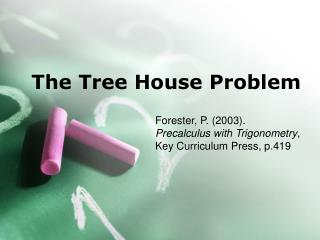DownloadDownload PresentationThe Tree House Problem

The Tree House Problem

Télécharger la présentationThe Tree House Problem

- - - - - - - - - - - - - - - - - - - - - - - - - - - E N D - - - - - - - - - - - - - - - - - - - - - - - - - - -
Presentation Transcript

1. The Tree House Problem Forester, P. (2003). Precalculus with Trigonometry, Key Curriculum Press, p.419

2. Elmer is going to build a tree house in the backyard for the children to play in.

3. The yard is level. He uses one corner of the yard as the origin of a three dimensional coordinate system. The x- and y- axis run along the ground, and the x-axis is vertical. He find that the tree house will be at the point (x, y, z) = (30, 55, 17) where the dimensions are in feet. 1. Sketch the coordinate axes and the point (30, 55,17).

4. East

5. North

6. Up

7. Write the position vector to the tree house. How high is the tree house above the group? How far is the tree house from the origin?

8. Answer: vector to the house (30, 55, 17), its 17 feet above the ground and to find how far from the origin: = 64.9153..

9. 3. A wire to be stretched from the tree house to the point (10,0,8) at the tope corner of the back door so that the children can slide messages down it. Write a vector representing the displacement from the tree house to the point on the back door.

10. 4. How long will the wire be?

11. The children slide a message down the wire. It gets stuck when it is only 30% of the way from the tree house to the back door. Write a vector representing the displacement from the tree house to the stuck message. How far along the wire did the message go before it got stuck?

12. Write the position vector of the stuck message Write the position vector of the stuck message. How high above the ground is the stuck message.